# Integrated Michaelis-Menten Equation

library(renz)
library(knitr)

## How many progress-curves do we need to estimate $$K_m$$ and $$V_{max}$$?

Suppose we have a Michaelian enzyme whose kinetic parameters we want to determine. To this end, a conventional approach is to find the initial rates at different concentrations of the substrate, to obtain, in this way, a dataset relating substrate concentration to rate. In this approach, a progress-curve for each initial substrate concentration assayed need to be built in order to calculate the initial rate as the slope of the tangent line to the curve at time 0.

For instance, let’s assay our enzyme at substrate concentrations 1, 2, 4, 8, 12, 20 and 30 mM.

At [S] = 1 mM: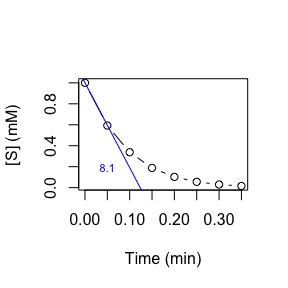At [S] = 2 mM: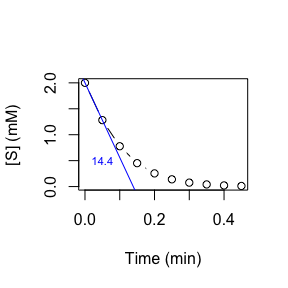At [S] = 4 mM: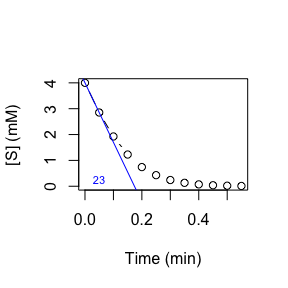At [S] = 8 mM: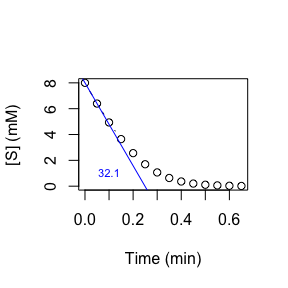At [S] = 12 mM: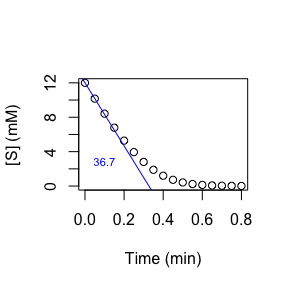At [S] = 20 mM: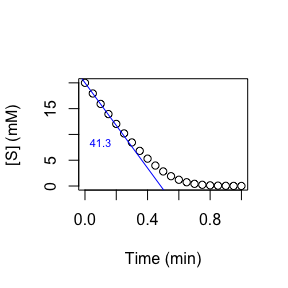At [S] = 30 mM: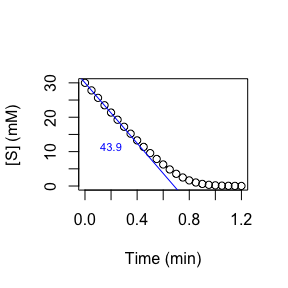Note that, in the current example, we have needed 7 progress-curves to collect the following dataset:

 [S] (mM) 1 2 4 8 12 20 30 v (mM/min) 8.1 14.4 23 32.1 36.7 41.3 43.9

Afterwards, we can proceed to estimate $$K_m$$ and $$V_{max}$$, for instance, by fitting our data to the Michaelis-Menten model:

dir.MM(Sv, unit_v = "mM/min")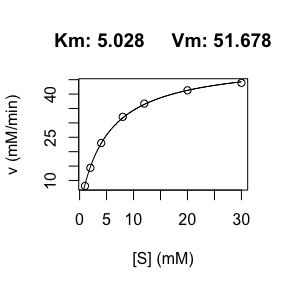#> $parameters #> Km Vm #> 5.028 51.678 #> #>$data
#>    S    v  fitted_v
#> 1  1  8.1  8.572993
#> 2  2 14.4 14.706318
#> 3  4 23.0 22.896766
#> 4  8 32.1 31.733497
#> 5 12 36.7 36.418605
#> 6 20 41.3 41.296148
#> 7 30 43.9 44.260021

Although we have succeeded in our aim (estimating the kinetic parameters) we have needed 7 progress-curves. Wouldn’t be nice to be able get $$K_m$$ and $$V_{max}$$ from one single progress-curve? Well, that is precisely what the integrated Michaelis-Menten equation allow us.

## Integrating the Michaelis-Menten equation

Suppose that, starting from an initial concentration of substrate 1 mM, we monitor their evolution over time, obtaining the following data:

t (min) [S] (mM)
0.00 1.000
0.05 0.593
0.10 0.338
0.15 0.188
0.20 0.103
0.25 0.056
0.30 0.030
0.35 0.016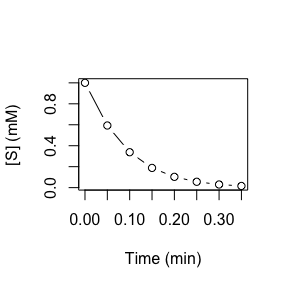Assuming that the substrate concentration drops as it is converted into a product by the direct unidirectional reaction catalyzed by our enzyme (this is only true when the reverse reaction is negligible, for example in hydrolysis reactions), and that the product has no effect on the catalyzed reaction rate, then we can relate the variation in the concentration of substrate over time integrating the velocity equation.

$\begin{equation} \tag{1} v = \frac{-d[S]}{dt} = V_{max} \frac{[S]}{[S] + K_m} \end{equation}$

$\begin{equation} \tag{2} \int_{S_o}^{S_t} \frac{[S] + K_m}{[S]} d[S] = -\int_{0}^t V_{max} dt \end{equation}$

$\begin{equation} \tag{3} \frac{1}{t} \ln \frac{S_o}{S_t} = - \frac{1}{K_m} \frac{(S_o - S_t)}{t} + \frac{V_{max}}{K_m} \end{equation}$

Equation (3) can be recognized as the equation of a line where the dependent variable is $$\frac{1}{t} \ln \frac{S_o}{S_t}$$ and the independent variable is $$\frac{(S_o - S_t)}{t}$$. Thus, if the data given in the table above are conveniently transformed, they can be fit to a line by least square.

The function int.MM() from the package renz takes as argument a dataframe where the first column is time and the second column is [S], and returns both the kinetic parameters and a dataframe with the transformed variable:

result <- int.MM(data)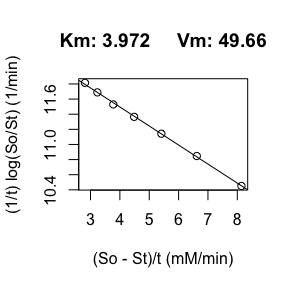## Some final considerations

In addition to saving us work, since a single progress-curve is enough to estimate the kinetic parameter, this method has an additional advantage: since the method does not employ initial rates, it avoid the bias introduced by underestimating initial rates (see doi: 10.1016/0307-4412(85)90008-1). Nevertheless, before using this approach, we have to make sure that the rate of the inverse reaction is negligible and that the product of the reaction does not affect the direct reaction rate.